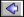back

# A Three-dimensional Map of the Milky Way Using 66,000 Mira Variable Stars

P. Iwanek, R. Poleski, S. Kozłowski, I. Soszyński, P. Pietrukowicz, M. Ban, J. Skowron, P. Mróz, M. Wrona, A. Udalski, M. K. Szymański, D. M. Skowron, K. Ulaczyk, M. Gromadzki, K. Rybicki, M. Ratajczak
ApJS, 264, 20 (arXiv:2212.00035)

We study the three-dimensional structure of the Milky Way using 65,981 Mira variable stars discovered by the Optical Gravitational Lensing Experiment (OGLE) survey. The spatial distribution of the Mira stars is analyzed with a model containing three barred components that include the X-shaped boxy component in the Galactic center (GC) and an axisymmetric disk. We take into account the distance uncertainties by implementing the Bayesian hierarchical inference method. The distance to the GC is R0 = 7.66 ± 0.01 (stat.) ± 0.39 (sys.) kpc, while the inclination of the major axis of the bulge to the Sun-GC line-of-sight is θ = 20.2℃ ± 0.6℃ (stat.) ± 0.7℃ (sys.). We present, for the first time, a detailed three-dimensional map of the Milky Way composed of young and intermediate-age stellar populations. Our analysis provides independent evidence for both the X-shaped bulge component and the flaring disk (being plausibly warped). We provide the complete dataset of properties of Miras that were used for calculations in this work. The table includes mean brightness and amplitudes in nine photometric bands (covering a range of wavelengths from 0.5 to 12 μm), photometric chemical type, estimated extinction, and calculated distance with its uncertainty for each Mira variable. The median distance accuracy to a Mira star is at the level of 6.6%.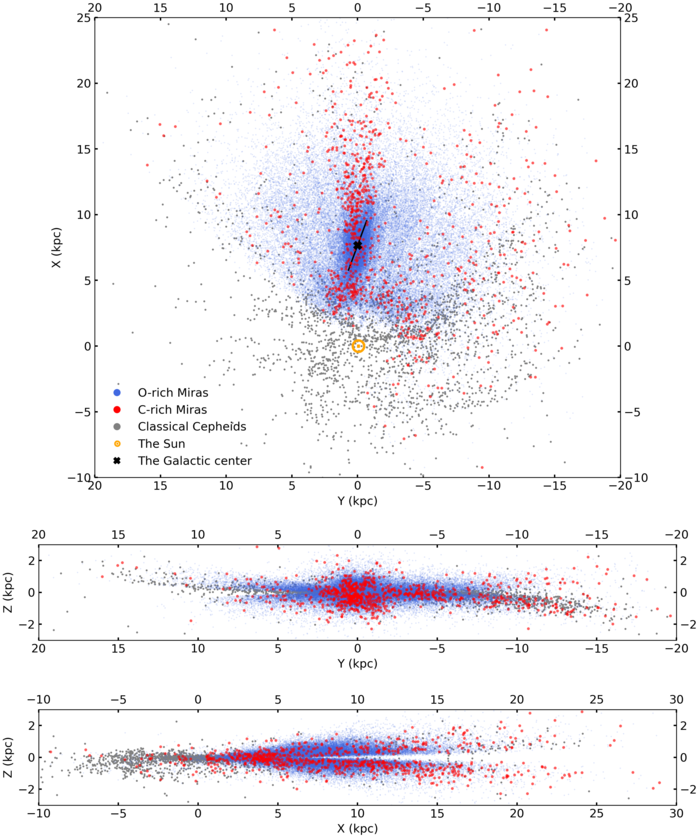Two-dimensional projections of the three-dimensional map of the Milky Way. With blue and red points, we show O-rich and C-rich Miras, respectively (the whole sample with distance accuracy better than 20%), while gray points represent Classical Cepheids analyzed by Skowron et al. (2019a). We marked the GC with a black cross (XGC = 7.66 kpc, YGC = -0.01 kpc), while we marked the solar system with an orange open circle with a center dot symbol. The black line along the bar shows the slope of the bar, i.e., θ angle (θ = 20.2℃). Top plot: Y–X projection of the Milky Way. Middle plot: Y–Z projection of the Milky Way. Bottom plot: X–Z projection of the Milky Way.

For detailed analysis of the Galactic bulge structure we use Sormani et al. (2022) model, which describes a three-dimensional density in the Cartesian coordinates and is composed of three barred components and an axisymmetric disk. The authors obtained this model by fitting a multicomponent parametric density distribution to a made-to-measure N-body model of Portail et al. (2017). The latter model was proposed because it accurately represents observations, both the density data and the kinematics of stars in the center of the Galaxy.

We fit Sormani et al. (2022) model to the Mira-type variables distribution by implementing the Bayesian hierarchical inference method to account for the distance uncertainties. This approach is commonly used for mapping non-linear phenomena where inferences are made about a population based on many individual observations arranged by levels. In this case, the distances of Mira variables are known with an uncertainty of about 10%, which constituted the second level of the Bayesian model.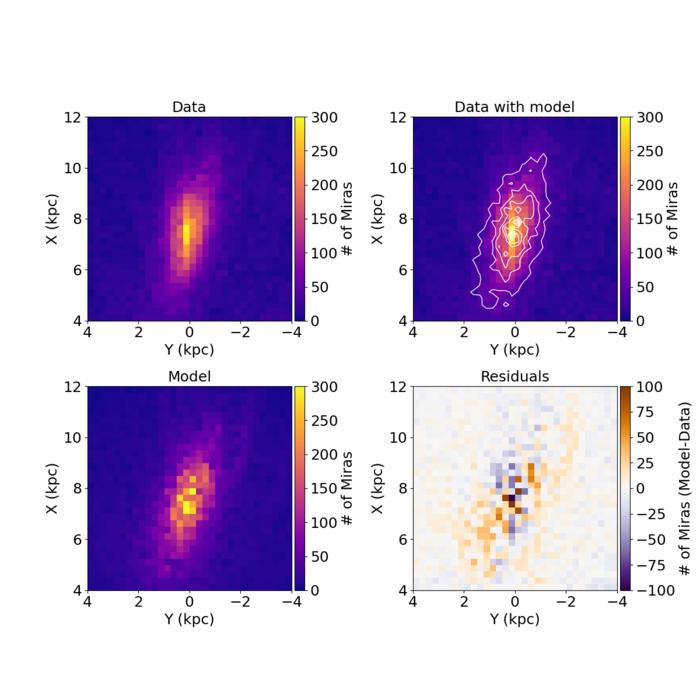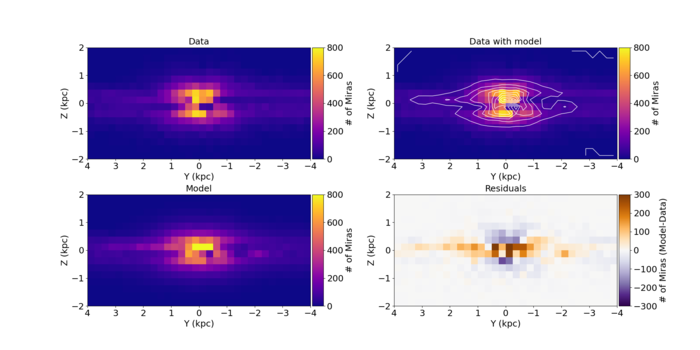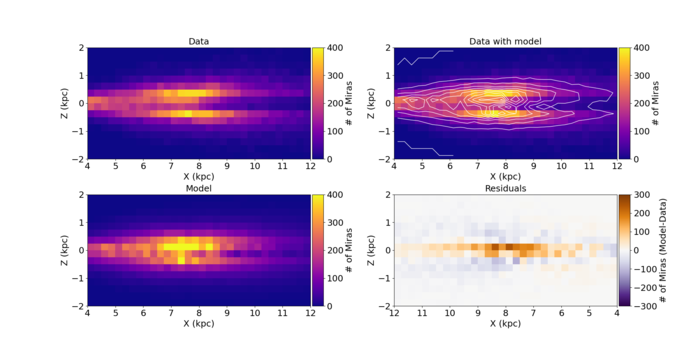From the top: the Y–X, Y–Z, and X–Z projections of the three-dimensional distribution of Miras and the fitted model. Miras are summed along the (from the top) Z–, X–, and Y–coordinate in each bin, respectively. In each Figure, the top left plot presents Miras used in the final fit, the top right plot presents the model of the bar (white contours) on top of the data, the bottom left plot presents the model of the bar, and finally the bottom right plot presents the difference between the model and Mira distributions, i.e., residuals. The model shows the same number of stars as the number of analyzed Miras.

The full machine-readable table with data used in this paper is available to the astronomical community from the OGLE Internet Archive (https://www.astrouw.edu.pl/ogle/ogle4/MILKY_WAY_3D_MAP/) or through the Zenodo (https://doi.org/10.5281/zenodo.7472598).

PLEASE cite the following paper when using the data or referring to these OGLE results:
Iwanek et al., 2023, ApJS, 264, 20 (DOI, arXiv:2212.00035)